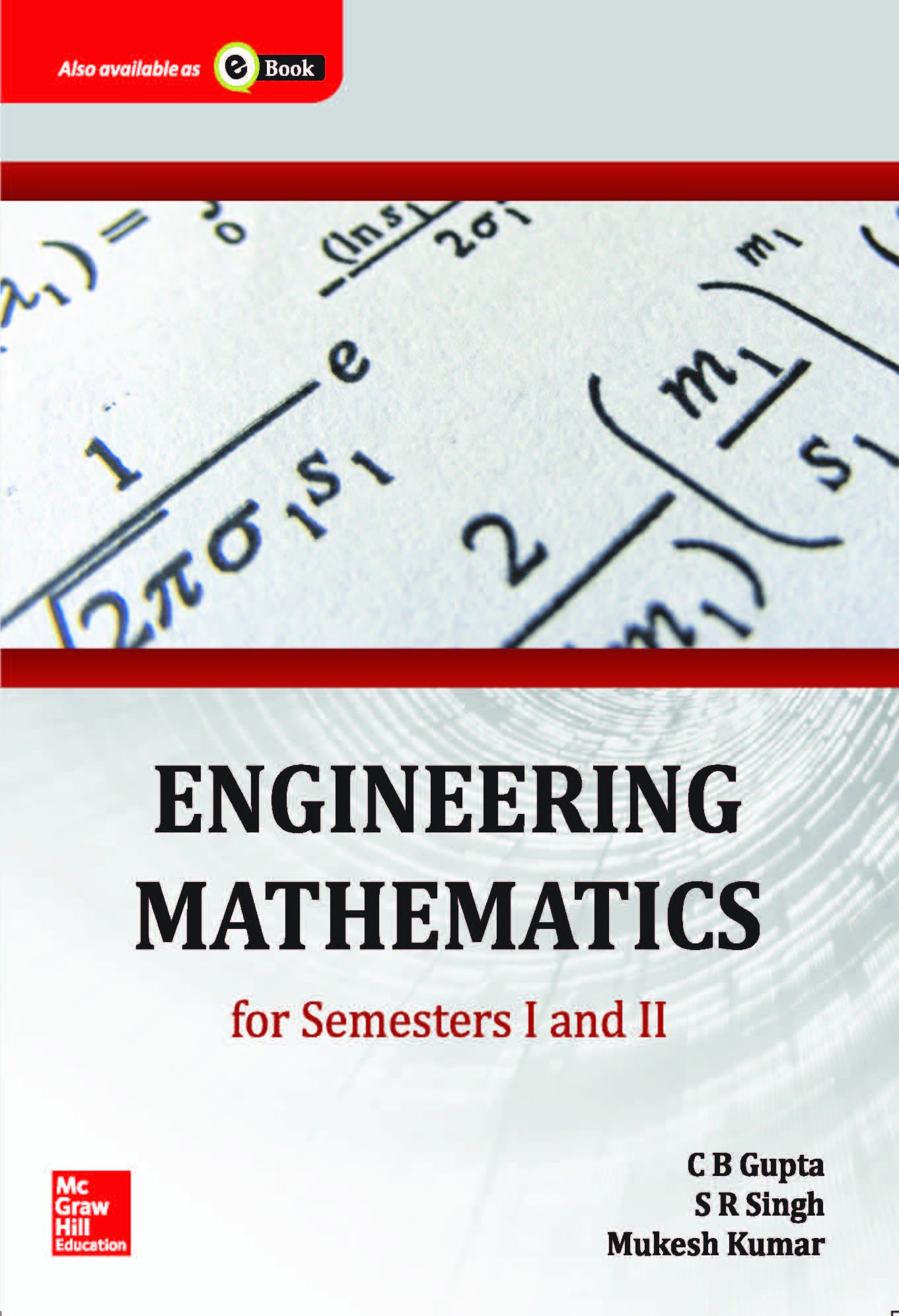# Engineering Mathematics for Semester I and IIPrice: ₹575.00
(as of Dec 08,2021 12:55:10 UTC – Details)The textbook on Engineering Mathematics has been created to provide an exposition of essential tools of engineering mathematics which forms the core of all branches of engineering – from aerospace engineering to electronics and from mechanical engineering to computer science – because it is believed that as engineering evolves and develops, mathematics forms the common foundation of all new disciplines.

Book Features:

• Problems derived from actual industrial situations presented with solutions
• Introduction to Infinite series, Fourier series, Laplace Transform, Differential and Integral Calculus with reference to applications in the field of engineering
• Pedagogy
• Solved examples: 700
• Drill and Practice problems: 1100
• Illustrations: 350

• Chapter 1: Matrix Algebra
• Chapter 2: Differential Calculus
• Chapter 3: Partial Differentiation
• Chapter 4: Maxima and Minima
• Chapter 5: Integral Calculus
• Chapter 6: Special Functions
• Chapter 7: Vector Differential and Integral Calculus
• Chapter 8: Infinite Series
• Chapter 9: Fourier Series
• Chapter 10: Differential Equations
• Chapter 11: Linear Differential Equations of Higher Order with Constant Coefficients
• Chapter 12: Solution of second order linear differential equations with variable coefficients Chapter 13: Series Solution
• Chapter 14: Partial Differential Equations
• Chapter 15: Application of Partial Differential Equations
• Chapter 16: Laplace Transform
• Appendix: Basic Formulae and Concepts

Scroll to Top